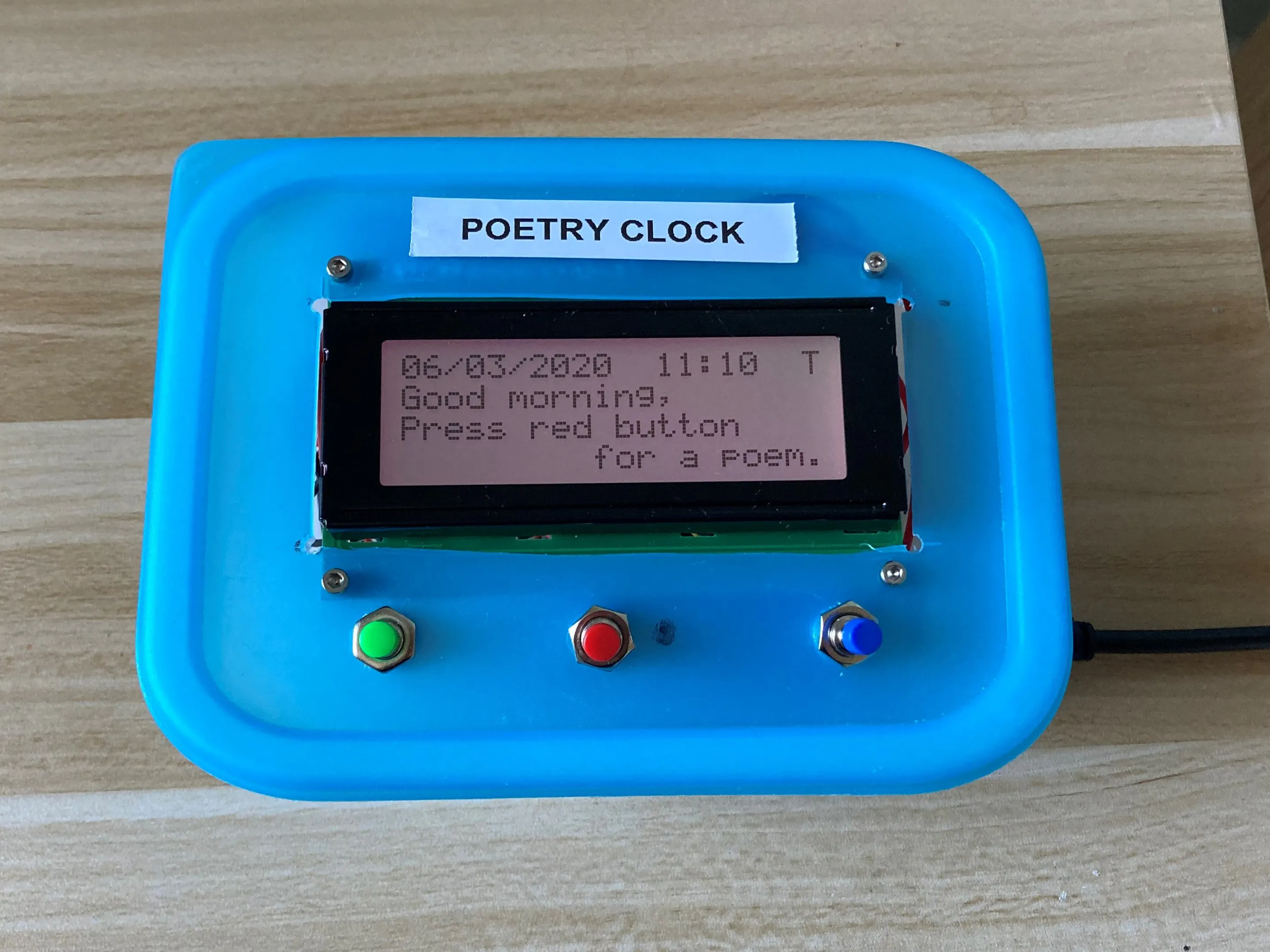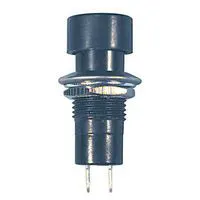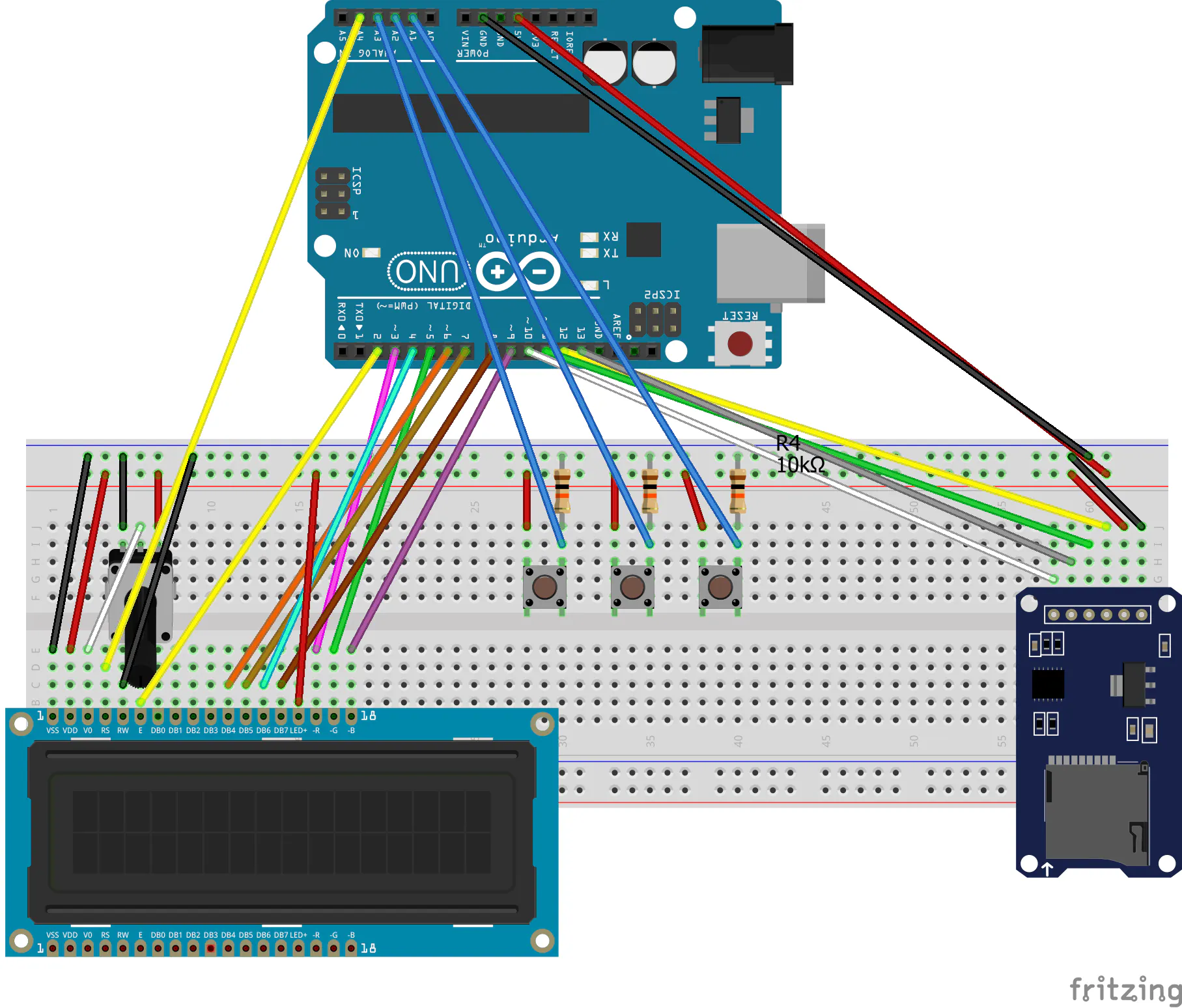LCD Poetry Clock

A 20x4 RGB LCD clock that can display over 500 poems using Arduino and an SD card reader.

IntermediateFull instructions provided1,928Things used in this project

Hardware components

 Adafruit 20 x 4 RGB LCD Display
×1Resistor 10k ohm
×3Pushbutton Switch, Momentary
×3
×1Arduino UNO
×1

SchematicsCode

Poetry Clock Code

C/C++
The Arduino Code for the Poetry Clock. Note you will need to download the SD fat library (version 1.1.4) to run this code. Download it here: https://github.com/greiman/SdFat
// SPI library to communicate with SD card
#include <SPI.h>

// SD card chip select pin.
const byte chipSelect = 10;

// File system object. Make SdFat compatible with SD.
#include <SdFat.h>
SdFat SD;

// Directory file.
File root;
// Text file
File entry;
//number of files in root
int rootFileCount = 0;

// initialize the LCD library by associating any LCD interface pin
// with the arduino pin number it is connected to
#include <LiquidCrystal.h>
const int rs = A4, en = 2, d4 = 6, d5 = 7, d6 = 4, d7 = 8;
LiquidCrystal lcd(rs, en, d4, d5, d6, d7);
// number of LCD columns and rows
const byte lcdCols = 20;
const byte lcdRows = 4;
// colour pins of LCD display
const byte redLed = 3;
const byte greenLed = 5;
const byte blueLed = 9;
// colour values of the backlight
int red = 30;
int green = 160;
int blue = 40;
// brightness of LCD display
int brightness = 180; // you can change the overall brightness by range 0 -> 255

//operating mode switch pin
const byte switchPin = A1;
int switchState;

// operating mode;
bool mode2 = false;

// timekeeping variables
int currentMin = 0;
int currentHour = 0;
int currentDay = 1;
int currentMonth = 1;
int currentYear = 2020;
int daysinMonth[] = {31,28,31,30,31,30,31,31,30,31,30,31};
unsigned long previousTime = 0;

// time switch pin
const byte timePin = A2;
int timeState;
// select switch pin
const byte selectPin = A3;
int selectState;
int selectCounter = 0;
char selectToken = 'T';

// determine whether to display poem of the day
bool potd = false;
// the potd number
int potd_n;
// index of poetry file to read on SD card
int threshold;

void setup() {
Serial.begin(9600);
// Wait for USB Serial
while (!Serial) {
SysCall::yield();
}
Serial.println("Serial initialized. Initializing LCD display...");
// set up the LCD's number of columns and rows:
lcd.begin(lcdCols, lcdRows);
// turn off LCD autoscrolling
lcd.noAutoscroll();
// set up LCD backlight pins
pinMode(redLed, OUTPUT);
pinMode(greenLed, OUTPUT);
pinMode(blueLed, OUTPUT);
// set up switch pins
pinMode(switchPin, INPUT);
pinMode(timePin, INPUT);
pinMode(selectPin, INPUT);
Serial.println("LCD initialized. Initializing SD card...");
// Initialize at the highest speed supported by the board that is
// not over 50 MHz. Try a lower speed if SPI errors occur.
delay(1000);
while (!SD.begin(chipSelect, SD_SCK_MHZ(50))) {
SD.initErrorHalt();
}
Serial.println("SD card initialized. Opening root directory...");
if (!root.open("/")) {
Serial.println("Opening root directory failed.");
}
//Serial.println("Root directory opened. Counting files...");
while (root.openNextFile()) {
rootFileCount++;
}
Serial.print(rootFileCount);
Serial.println(" files counted.");
// generate a seed for the RNG and random starting colours
// initialise potd_n value
potd_n = random(rootFileCount);
// set the LCD display backlight based on currentTime
setBacklight(red,green,blue);
}

void loop() {
// time keeping
unsigned long currentTime = millis();
// calculate minutes, hours, days, months and years
if (currentTime - previousTime > 60000){
currentMin++;
previousTime = currentTime;
}
if (currentMin >= 60){
currentMin = 0;
currentHour ++;
}
if (currentHour >= 24){
currentHour = 0;
currentDay ++;
potd_n = random(rootFileCount);
}
if (currentDay >= daysinMonth[currentMonth]){
currentDay = 1;
currentMonth ++;
}
if (currentMonth >= 13){
currentMonth = 1;
currentYear ++;
}
// read the select state to determine what time parameter is to be changed
if (selectState == HIGH){
selectCounter ++;
if (selectCounter == 5){
selectCounter = 0;
}
switch(selectCounter){
case 0:
selectToken = 'T';
break;
case 1:
selectToken = 'H';
break;
case 2:
selectToken = 'D';
break;
case 3:
selectToken = 'M';
break;
case 4:
selectToken = 'Y';
}
delay(250);
}
// read the time state to determine time
if (timeState == HIGH){
switch(selectCounter){
case 0:
currentMin ++;
break;
case 1:
currentHour ++;
break;
case 2:
currentDay ++;
break;
case 3:
currentMonth ++;
break;
case 4:
currentYear ++;
}
delay(250);
}
// read the switch state to determine operating mode
if (switchState == HIGH){
mode2 = true;
}
if (mode2 == true){
//
// MODE 2: RANDOM POEM
// display a random poem from the SD card line by line
//
// initialise backlight with random r,g,b values;
red = random(256);
green = random(256);
blue = random(256);
setBacklight(red,green,blue);
//if (!SD.begin(chipSelect, SD_SCK_MHZ(50))) {
//  SD.initErrorHalt();
//}
//if (!root.open("/")) {
//  Serial.println("Opening root directory failed.");
//}
// rewind root directory to begining
root.rewindDirectory();
// generate random number up to file count and open the corresponding file
if (potd == false){
threshold = random(0,rootFileCount);
// use potd_n as the threshold value
} else {
threshold = potd_n;
}
int i = 0;
while (i < threshold) {
entry = root.openNextFile();
i++;
}
// arrays with characters to display on each row of lcd screen
char row0[lcdCols];
char row1[lcdCols];
char row2[lcdCols];
char row3[lcdCols];
// initialise as empty otherwise problems will occur when reading first line
memset(row0, ' ', lcdCols);
memset(row1, ' ', lcdCols);
memset(row2, ' ', lcdCols);
memset(row3, ' ', lcdCols);
// read the file byte by byte and display it on the LCD screen
byte b = 0;
byte le;
bool leFlag = false;
while (entry.available()){
//if the next byte is a carriage return
if (entry.peek() == 13){
leFlag = true;
//ignore carriage return and line end symbols
}
else {
// ignore any non basic ascii symbols
while (entry.peek() < 32 || entry.peek() > 126) {
}
//first row
if (b < lcdCols){
// prevent words from splitting over lines
if (b == lcdCols - 1 && entry.peek() != ' '){
//find the last instance of space in row0
le = lineEnd(row0,lcdCols);
//shift all text after the last space to the row beneath
for (byte c = le; c < lcdCols; c++){
row1[c-le] = row0[c];
row0[c] = ' ';
}
//update the index position to account for the shift;
b = 2 * lcdCols - 1 - le;
}
//second row
} else if (b < lcdCols * 2) {
// prevent words from splitting over lines
if (b == lcdCols * 2 - 1 && entry.peek() != ' '){
//find the last instance of space in row1
le = lineEnd(row1,lcdCols);
//shift all text after the last space to the row beneath
for (byte c = le; c < lcdCols; c++){
row2[c-le] = row1[c];
row1[c] = ' ';
}
//update the index position to account for the shift;
b = 3 * lcdCols - 1 - le;
}
//third row
} else if (b < lcdCols * 3) {
// prevent words from splitting over lines
if (b == lcdCols * 3 - 1 && entry.peek() != ' '){
//find the last instance of space in row2
le = lineEnd(row2,lcdCols);
//shift all text after the last space to the row beneath
for (byte c = le; c < lcdCols; c++){
row3[c-le] = row2[c];
row2[c] = ' ';
}
//update the index position to account for the shift;
b = 4 * lcdCols - 1 - le;
}
//fourth row
} else if (b < lcdCols * 4) {
}
b++;
}
// write the row bytes to the display
if (b > lcdCols * 4 || leFlag == true) {
lcd.clear();
lcd.home();
lcd.write(row0);
lcd.setCursor(0,1);
lcd.write(row1);
lcd.setCursor(0,2);
lcd.write(row2);
lcd.setCursor(0,3);
lcd.write(row3);
// time to wait between displaying lines
delay(2500);
memset(row0, ' ', lcdCols);
memset(row1, ' ', lcdCols);
memset(row2, ' ', lcdCols);
memset(row3, ' ', lcdCols);
b = 0;
leFlag = false;
}
}
// clear the screen and reset the cursor
lcd.clear();
lcd.home();
entry.close();
mode2 = false;
}
else {
//
// MODE 1: Clock
// LCD display functions as a clock, displaying time and date.
// Time and date are adjustable using PTM switches.
// Every 15 minutes a random poem is displayed.
//
lcd.home();
//Print time and date
printDate(currentDay,currentMonth,currentYear);
lcd.print("  ");
printTime(currentMin,currentHour);
lcd.print("  ");
// print character to show which parameter is changed by timeSwitch;
lcd.print(selectToken);
lcd.setCursor(0,1);
if(currentHour >= 4 and currentHour < 12){
lcd.print("Good morning,  ");
}else if (currentHour >= 12 and currentHour < 19){
lcd.print("Good afternoon,");
} else {
lcd.print("Good evening,  ");
}
lcd.setCursor(0,2);
lcd.print("Press red button");
lcd.setCursor(0,3);
lcd.print("         for a poem.");
// display poem of the day every 15 minutes
if (currentMin % 15 == 0 and potd == false){
mode2 = true;
potd = true;
// untill the minute is up pressing the red button will read poem of the day
} else if (currentMin % 15 != 0){
potd = false;
}
}
}
// determine the position of where to break the display in a row of characters
byte lineEnd(char row[], byte rowLength){
byte le = 0;
for (byte c = 0; c < rowLength; c++){
if (row[c] == ' '){
le = c;
}
}
return le;
}
// set the LED backlight
void setBacklight(uint8_t r, uint8_t g, uint8_t blue) {
// normalize the red LED - its brighter than the rest!
r = map(r, 0, 255, 0, 100);
g = map(g, 0, 255, 0, 150);

r = map(r, 0, 255, 0, brightness);
g = map(g, 0, 255, 0, brightness);
blue = map(blue, 0, 255, 0, brightness);

// common anode so invert!
r = map(r, 0, 255, 255, 0);
g = map(g, 0, 255, 255, 0);
blue = map(blue, 0, 255, 255, 0);
Serial.print("R = "); Serial.print(r, DEC);
Serial.print(" G = "); Serial.print(g, DEC);
Serial.print(" B = "); Serial.println(blue, DEC);
analogWrite(redLed, r);
analogWrite(greenLed, g);
analogWrite(blueLed, blue);
}
//print time (hours:minutes)
void printTime(int currentMin,int currentHour){
if (currentHour < 10){
lcd.print("0");
}
lcd.print(currentHour);
lcd.print(":");
if (currentMin < 10){
lcd.print("0");
}
lcd.print(currentMin);
}
//print date(day:month:year)
void printDate(int currentDay, int currentMonth, int currentYear){
if (currentDay < 10){
lcd.print("0");
}
lcd.print(currentDay);
lcd.print("/");
if (currentMonth < 10){
lcd.print("0");
}
lcd.print(currentMonth);
lcd.print("/");
lcd.print(currentYear);
}

Poetry Clock Files

Zip file containing 526 poems used in the clock and the python script used to download them.